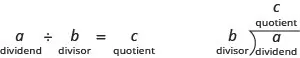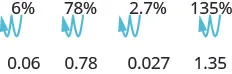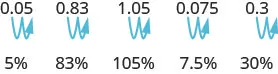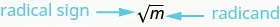Intermediate Algebra 2e

# 1.4Decimals

### Learning Objectives

By the end of this section, you will be able to:

• Round decimals
• Multiply and divide decimals
• Convert decimals, fractions, and percents
• Simplify expressions with square roots
• Identify integers, rational numbers, irrational numbers, and real numbers
• Locate fractions and decimals on the number line

### Be Prepared 1.4

A more thorough introduction to the topics covered in this section can be found in the Elementary Algebra 2e chapter, Foundations.

### Round Decimals

Decimals are another way of writing fractions whose denominators are powers of ten.

$0.1=110is “one tenth” 0.01=1100is “one hundredth” 0.001=11000is “one thousandth” 0.0001=110,000is “one ten-thousandth”0.1=110is “one tenth” 0.01=1100is “one hundredth” 0.001=11000is “one thousandth” 0.0001=110,000is “one ten-thousandth”$

Just as in whole numbers, each digit of a decimal corresponds to the place value based on the powers of ten. Figure 1.6 shows the names of the place values to the left and right of the decimal point.

Figure 1.6

When we work with decimals, it is often necessary to round the number to the nearest required place value. We summarize the steps for rounding a decimal here.

### How To

#### Round decimals.

1. Step 1. Locate the given place value and mark it with an arrow.
2. Step 2. Underline the digit to the right of the place value.
3. Step 3.
Is the underlined digit greater than or equal to 5?
• Yes: add 1 to the digit in the given place value.
• No: do not change the digit in the given place value
4. Step 4. Rewrite the number, deleting all digits to the right of the rounding digit.

### Example 1.34

Round 18.379 to the nearest hundredth tenth whole number.

### Try It 1.67

Round $6.5826.582$ to the nearest hundredth tenth whole number.

### Try It 1.68

Round $15.217515.2175$ to the nearest thousandth hundredth tenth.

To add or subtract decimals, we line up the decimal points. By lining up the decimal points this way, we can add or subtract the corresponding place values. We then add or subtract the numbers as if they were whole numbers and then place the decimal point in the sum.

### How To

1. Step 1. Determine the sign of the sum or difference.
2. Step 2. Write the numbers so the decimal points line up vertically.
3. Step 3. Use zeros as placeholders, as needed.
4. Step 4. Add or subtract the numbers as if they were whole numbers. Then place the
decimal point in the answer under the decimal points in the given numbers.
5. Step 5. Write the sum or difference with the appropriate sign.

### Example 1.35

Add or subtract: $−23.5−41.38−23.5−41.38$ $14.65−20.14.65−20.$

### Try It 1.69

Add or subtract: $−4.8−11.69−4.8−11.69$ $9.58−10.9.58−10.$

### Try It 1.70

Add or subtract: $−5.123−18.47−5.123−18.47$ $37.42−50.37.42−50.$

### Multiply and Divide Decimals

When we multiply signed decimals, first we determine the sign of the product and then multiply as if the numbers were both positive. We multiply the numbers temporarily ignoring the decimal point and then count the number of decimal points in the factors and that sum tells us the number of decimal places in the product. Finally, we write the product with the appropriate sign.

### How To

#### Multiply decimals.

1. Step 1. Determine the sign of the product.
2. Step 2. Write in vertical format, lining up the numbers on the right. Multiply the numbers as if they were whole numbers, temporarily ignoring the decimal points.
3. Step 3. Place the decimal point. The number of decimal places in the product is the sum of
the number of decimal places in the factors.
4. Step 4. Write the product with the appropriate sign.

### Example 1.36

Multiply: $(−3.9)(4.075).(−3.9)(4.075).$

### Try It 1.71

Multiply: $−4.5(6.107).−4.5(6.107).$

### Try It 1.72

Multiply: $−10.79(8.12).−10.79(8.12).$

Often, especially in the sciences, you will multiply decimals by powers of 10 (10, 100, 1000, etc). If you multiply a few products on paper, you may notice a pattern relating the number of zeros in the power of 10 to number of decimal places we move the decimal point to the right to get the product.

### How To

#### Multiply a decimal by a power of ten.

1. Step 1. Move the decimal point to the right the same number of places as the
number of zeros in the power of 10.
2. Step 2. Add zeros at the end of the number as needed.

### Example 1.37

Multiply: 5.63 by 10 100 1000.

### Try It 1.73

Multiply 2.58 by 10 100 1000.

### Try It 1.74

Multiply 14.2 by 10 100 1000.

Just as with multiplication, division of signed decimals is very much like dividing whole numbers. We just have to figure out where the decimal point must be placed and the sign of the quotient. When dividing signed decimals, first determine the sign of the quotient and then divide as if the numbers were both positive. Finally, write the quotient with the appropriate sign.

We review the notation and vocabulary for division:We’ll write the steps to take when dividing decimals for easy reference.

### How To

#### Divide decimals.

1. Step 1. Determine the sign of the quotient.
2. Step 2. Make the divisor a whole number by “moving” the decimal point all the way to the right. “Move” the decimal point in the dividend the same number of places—adding zeros as needed.
3. Step 3. Divide. Place the decimal point in the quotient above the decimal point in the dividend.
4. Step 4. Write the quotient with the appropriate sign.

### Example 1.38

Divide: $−25.65÷(−0.06).−25.65÷(−0.06).$

### Try It 1.75

Divide: $−23.492÷(−0.04).−23.492÷(−0.04).$

### Try It 1.76

Divide: $−4.11÷(−0.12).−4.11÷(−0.12).$

### Convert Decimals, Fractions, and Percents

In our work, it is often necessary to change the form of a number. We may have to change fractions to decimals or decimals to percent.

We convert decimals into fractions by identifying the place value of the last (farthest right) digit. In the decimal $0.03.0.03.$ the 3 is in the hundredths place, so 100 is the denominator of the fraction equivalent to 0.03.

$0.03=31000.03=3100$

The steps to take to convert a decimal to a fraction are summarized in the procedure box.

### How To

#### Convert a decimal to a proper fraction and a fraction to a decimal.

1. Step 1. To convert a decimal to a proper fraction, determine the place value of the final digit.
2. Step 2.
Write the fraction.
• numerator—the “numbers” to the right of the decimal point
• denominator—the place value corresponding to the final digit
3. Step 3. To convert a fraction to a decimal, divide the numerator of the fraction by the denominator of the fraction.

### Example 1.39

Write: $0.3740.374$ as a fraction $−58−58$ as a decimal.

### Try It 1.77

Write: $0.2340.234$ as a fraction $−78−78$ as a decimal.

### Try It 1.78

Write: $0.0240.024$ as a fraction $−38−38$ as a decimal.

A percent is a ratio whose denominator is 100. Percent means per hundred. We use the percent symbol, %, to show percent. Since a percent is a ratio, it can easily be expressed as a fraction. Percent means per 100, so the denominator of the fraction is 100. We then change the fraction to a decimal by dividing the numerator by the denominator. After doing this many times, you may see the pattern.

To convert a percent number to a decimal number, we move the decimal point two places to the left.To convert a decimal to a percent, remember that percent means per hundred. If we change the decimal to a fraction whose denominator is 100, it is easy to change that fraction to a percent. After many conversions, you may recognize the pattern.

To convert a decimal to a percent, we move the decimal point two places to the right and then add the percent sign.### How To

#### Convert a percent to a decimal and a decimal to a percent.

1. Step 1. To convert a percent to a decimal, move the decimal point two places to the left after removing the percent sign.
2. Step 2. To convert a decimal to a percent, move the decimal point two places to the right and then add the percent sign.

### Example 1.40

Convert each:

percent to a decimal: 62%, 135%, and 13.7%.

decimal to a percent: 0.51, 1.25, and 0.093.

### Try It 1.79

Convert each:

percent to a decimal: 9%, 87%, and 3.9%.

decimal to a percent: 0.17, 1.75, and 0.0825.

### Try It 1.80

Convert each:

percent to a decimal: 3%, 91%, and 8.3%.

decimal to a percent: 0.41, 2.25, and 0.0925.

### Simplify Expressions with Square Roots

Remember that when a number $nn$ is multiplied by itself, we write $n2n2$ and read it “$nn$ squared.” The result is called the square of a number n. For example, $8282$ is read “8 squared” and 64 is called the square of 8. Similarly, 121 is the square of 11 because $112112$ is 121. It will be helpful to learn to recognize the perfect square numbers.

### Square of a number

If $n2=m,n2=m,$ then m is the square of n.

What about the squares of negative numbers? We know that when the signs of two numbers are the same, their product is positive. So the square of any negative number is also positive.

$(−3)2=9(−8)2=64(−11)2=121(−15)2=225(−3)2=9(−8)2=64(−11)2=121(−15)2=225$

Because $102=100,102=100,$ we say 100 is the square of 10. We also say that 10 is a square root of 100. A number whose square is m is called a square root of a number m.

### Square Root of a Number

If $n2=m,n2=m,$ then n is a square root of m.

Notice $(−10)2=100(−10)2=100$ also, so $−10−10$ is also a square root of 100. Therefore, both 10 and $−10−10$ are square roots of 100. So, every positive number has two square roots—one positive and one negative. The radical sign, $mm$, denotes the positive square root. The positive square root is called the principal square root. When we use the radical sign that always means we want the principal square root.

### Square Root Notation

$mm$ is read “the square root of $mm$.”If $m=n2,m=n2,$ then $m=n,m=n,$ for $n≥0.n≥0.$

The square root of m, $m,m,$ is the positive number whose square is m.

We know that every positive number has two square roots and the radical sign indicates the positive one. We write $100=10.100=10.$ If we want to find the negative square root of a number, we place a negative in front of the radical sign. For example, $−100=−10.−100=−10.$ We read $−100−100$ as “the opposite of the principal square root of 10.”

### Example 1.41

Simplify: $2525$ $121121$ $−144.−144.$

### Try It 1.81

Simplify: $3636$ $169169$ $−225.−225.$

### Try It 1.82

Simplify: $1616$ $196196$ $−100.−100.$

### Identify Integers, Rational Numbers, Irrational Numbers, and Real Numbers

We have already described numbers as counting numbers, whole numbers, and integers. What is the difference between these types of numbers? Difference could be confused with subtraction. How about asking how we distinguish between these types of numbers?

$Counting numbers1,2,3,4,….. Whole numbers0,1,2,3,4,…. Integers….−3,−2,−1,0,1,2,3,…. Counting numbers1,2,3,4,….. Whole numbers0,1,2,3,4,…. Integers….−3,−2,−1,0,1,2,3,….$

What type of numbers would we get if we started with all the integers and then included all the fractions? The numbers we would have form the set of rational numbers. A rational number is a number that can be written as a ratio of two integers.

In general, any decimal that ends after a number of digits (such as 7.3 or $−1.2684−1.2684$) is a rational number. We can use the reciprocal (or multiplicative inverse) of the place value of the last digit as the denominator when writing the decimal as a fraction. The decimal for $1313$ is the number $0.3–.0.3–.$ The bar over the 3 indicates that the number 3 repeats infinitely. Continuously has an important meaning in calculus. The number(s) under the bar is called the repeating block and it repeats continuously.

Since all integers can be written as a fraction whose denominator is 1, the integers (and so also the counting and whole numbers. are rational numbers.

Every rational number can be written both as a ratio of integers $pq,pq,$ where p and q are integers and $q≠0,q≠0,$ and as a decimal that stops or repeats.

### Rational Number

A rational number is a number of the form $pq,pq,$ where p and q are integers and $q≠0.q≠0.$

Its decimal form stops or repeats.

Are there any decimals that do not stop or repeat? Yes! The number $ππ$ (the Greek letter pi, pronounced “pie”), which is very important in describing circles, has a decimal form that does not stop or repeat. We use three dots (…) to indicate the decimal does not stop or repeat.

$π=3.141592654...π=3.141592654...$

The square root of a number that is not a perfect square is a decimal that does not stop or repeat.

A numbers whose decimal form does not stop or repeat cannot be written as a fraction of integers. We call this an irrational number.

### Irrational Number

An irrational number is a number that cannot be written as the ratio of two integers.

Its decimal form does not stop and does not repeat.

Let’s summarize a method we can use to determine whether a number is rational or irrational.

### Rational or Irrational

If the decimal form of a number

• repeats or stops, the number is a rational number.
• does not repeat and does not stop, the number is an irrational number.

We have seen that all counting numbers are whole numbers, all whole numbers are integers, and all integers are rational numbers. The irrational numbers are numbers whose decimal form does not stop and does not repeat. When we put together the rational numbers and the irrational numbers, we get the set of real numbers.

### Real Number

A real number is a number that is either rational or irrational.

Later in this course we will introduce numbers beyond the real numbers. Figure 1.7 illustrates how the number sets we’ve used so far fit together.

Figure 1.7 This chart shows the number sets that make up the set of real numbers.

Does the term “real numbers” seem strange to you? Are there any numbers that are not “real,” and, if so, what could they be? Can we simplify $−25?−25?$ Is there a number whose square is $−25?−25?$

$()2=−25?()2=−25?$

None of the numbers that we have dealt with so far has a square that is $−25.−25.$ Why? Any positive number squared is positive. Any negative number squared is positive. So we say there is no real number equal to $−25.−25.$ The square root of a negative number is not a real number.

### Example 1.42

Given the numbers $−7,145,8,5,5.9,−64,−7,145,8,5,5.9,−64,$ list the whole numbers integers rational numbers irrational numbers real numbers.

### Try It 1.83

Given the numbers $−3,−2,0.3–,95,4,49,−3,−2,0.3–,95,4,49,$ list the whole numbers integers rational numbers
irrational numbers real numbers.

### Try It 1.84

Given numbers $−25,−38,−1,6,121,2.041975...,−25,−38,−1,6,121,2.041975...,$ list the whole numbers integers rational numbers irrational numbers real numbers.

### Locate Fractions and Decimals on the Number Line

We now want to include fractions and decimals on the number line. Let’s start with fractions and locate $15,−45,3,74,−92,−515,−45,3,74,−92,−5$ and $8383$ on the number line.

We’ll start with the whole numbers 3 and $−5−5$ because they are the easiest to plot. See Figure 1.8.

The proper fractions listed are $1515$ and $−45.−45.$ We know the proper fraction $1515$ has value less than one and so would be located between 0 and 1. The denominator is 5, so we divide the unit from 0 to 1 into 5 equal parts $15,25,35,45.15,25,35,45.$ We plot $15.15.$

Similarly, $−45−45$ is between 0 and $−1.−1.$ After dividing the unit into 5 equal parts we plot $−45.−45.$

Finally, look at the improper fractions $74,92,83.74,92,83.$ Locating these points may be easier if you change each of them to a mixed number.

$74=134−92=−41283=22374=134−92=−41283=223$

Figure 1.8 shows the number line with all the points plotted.

Figure 1.8

### Example 1.43

Locate and label the following on a number line: $4,34,−14,−3,65,−52,4,34,−14,−3,65,−52,$ and $73.73.$

### Try It 1.85

Locate and label the following on a number line: $−1,13,65,−74,92,5,−83.−1,13,65,−74,92,5,−83.$

### Try It 1.86

Locate and label the following on a number line: $−2,23,75,−74,72,3,−73.−2,23,75,−74,72,3,−73.$

Since decimals are forms of fractions, locating decimals on the number line is similar to locating fractions on the number line.

### Example 1.44

Locate on the number line: 0.4 $−0.74.−0.74.$

### Try It 1.87

Locate on the number line: $0.60.6$ $−0.25.−0.25.$

### Try It 1.88

Locate on the number line: $0.90.9$ $−0.75.−0.75.$

### Media

Access this online resource for additional instruction and practice with decimals.

### Section 1.4 Exercises

#### Practice Makes Perfect

Round Decimals

In the following exercises, round each number to the nearest hundredth tenth whole number.

239.

5.781

240.

1.638

241.

0.299

242.

0.697

243.

63.479

244.

84.281

In the following exercises, add or subtract.

245.

$−16.53 − 24.38 −16.53 − 24.38$

246.

$−19.47 − 32.58 −19.47 − 32.58$

247.

$−38.69 + 31.47 −38.69 + 31.47$

248.

$−29.83 + 19.76 −29.83 + 19.76$

249.

$72.5 − 100 72.5 − 100$

250.

$86.2 − 100 86.2 − 100$

251.

$91.75 − ( −10.462 ) 91.75 − ( −10.462 )$

252.

$94.69 − ( −12.678 ) 94.69 − ( −12.678 )$

253.

$55.01 − 3.7 55.01 − 3.7$

254.

$59.08 − 4.6 59.08 − 4.6$

255.

$2.51 − 7.4 2.51 − 7.4$

256.

$3.84 − 6.1 3.84 − 6.1$

Multiply and Divide Decimals

In the following exercises, multiply.

257.

$( 94.69 ) ( −12.678 ) ( 94.69 ) ( −12.678 )$

258.

$( −8.5 ) ( 1.69 ) ( −8.5 ) ( 1.69 )$

259.

$( −5.18 ) ( −65.23 ) ( −5.18 ) ( −65.23 )$

260.

$( −9.16 ) ( −68.34 ) ( −9.16 ) ( −68.34 )$

261.

$( 0.06 ) ( 21.75 ) ( 0.06 ) ( 21.75 )$

262.

$( 0.08 ) ( 52.45 ) ( 0.08 ) ( 52.45 )$

263.

$( 9.24 ) ( 10 ) ( 9.24 ) ( 10 )$

264.

$( 6.531 ) ( 10 ) ( 6.531 ) ( 10 )$

265.

$( 0.025 ) ( 100 ) ( 0.025 ) ( 100 )$

266.

$( 0.037 ) ( 100 ) ( 0.037 ) ( 100 )$

267.

$( 55.2 ) ( 1000 ) ( 55.2 ) ( 1000 )$

268.

$( 99.4 ) ( 1000 ) ( 99.4 ) ( 1000 )$

In the following exercises, divide. Round money monetary answers to the nearest cent.

269.

$117.25 ÷ 48 117.25 ÷ 48$

270.

$109.24 ÷ 36 109.24 ÷ 36$

271.

$1.44 ÷ ( −0.3 ) 1.44 ÷ ( −0.3 )$

272.

$−1.15 ÷ ( −0.05 ) −1.15 ÷ ( −0.05 )$

273.

$5.2 ÷ 2.5 5.2 ÷ 2.5$

274.

$14 ÷ 0.35 14 ÷ 0.35$

Convert Decimals, Fractions and Percents

In the following exercises, write each decimal as a fraction.

275.

$0.04 0.04$

276.

1.464

277.

$0.095 0.095$

278.

$−0.375 −0.375$

In the following exercises, convert each fraction to a decimal.

279.

$17 20 17 20$

280.

$17 4 17 4$

281.

$− 310 25 − 310 25$

282.

$− 18 11 − 18 11$

In the following exercises, convert each percent to a decimal.

283.

$71 % 71 %$

284.

$150 % 150 %$

285.

$39.3 % 39.3 %$

286.

$7.8 % 7.8 %$

In the following exercises, convert each decimal to a percent.

287.

$1.56 1.56$

288.

3

289.

$0.0625 0.0625$

290.

$2.254 2.254$

Simplify Expressions with Square Roots

In the following exercises, simplify.

291.

$64 64$

292.

$169 169$

293.

$144 144$

294.

$− 4 − 4$

295.

$− 100 − 100$

296.

$− 121 − 121$

Identify Integers, Rational Numbers, Irrational Numbers, and Real Numbers

In the following exercises, list the whole numbers, integers, rational numbers, irrational numbers, real numbers for each set of numbers.

297.

$−8 , 0 , 1.95286... , 12 5 , 36 , 9 −8 , 0 , 1.95286... , 12 5 , 36 , 9$

298.

$−9 , −3 4 9 , − 9 , 0.4 09 — , 11 6 , 7 −9 , −3 4 9 , − 9 , 0.4 09 — , 11 6 , 7$

299.

$− 100 , −7 , − 8 3 , −1 , 0.77 , 3 1 4 − 100 , −7 , − 8 3 , −1 , 0.77 , 3 1 4$

300.

$−6 , − 5 2 , 0 , 0. 714285 ——— , 2 1 5 , 14 −6 , − 5 2 , 0 , 0. 714285 ——— , 2 1 5 , 14$

Locate Fractions and Decimals on the Number Line

In the following exercises, locate the numbers on a number line.

301.

$3 10 , 7 2 , 11 6 , 4 3 10 , 7 2 , 11 6 , 4$

302.

$7 10 , 5 2 , 13 8 , 3 7 10 , 5 2 , 13 8 , 3$

303.

$3 4 , − 3 4 , 1 2 3 , −1 2 3 , 5 2 , − 5 2 3 4 , − 3 4 , 1 2 3 , −1 2 3 , 5 2 , − 5 2$

304.

$2 5 , − 2 5 , 1 3 4 , −1 3 4 , 8 3 , − 8 3 2 5 , − 2 5 , 1 3 4 , −1 3 4 , 8 3 , − 8 3$

305.

$0.80.8$ $−1.25−1.25$

306.

$−0.9−0.9$ $−2.75−2.75$

307.

$−1.6−1.6$ $3.253.25$

308.

$3.13.1$ $−3.65−3.65$

#### Writing Exercises

309.

310.

When the Szetos sold their home, the selling price was 500% of what they had paid for the house 30 years ago. Explain what 500% means in this context.

311.

In your own words, explain the difference between a rational number and an irrational number.

312.

Explain how the sets of numbers (counting, whole, integer, rational, irrationals, reals) are related to each other.

#### Self Check

Use this checklist to evaluate your mastery of the objectives of this section.On a scale of 1-10, how would you rate your mastery of this section in light of your responses on the checklist? How can you improve this?

Order a print copy

As an Amazon Associate we earn from qualifying purchases.